# Data Collection for Mobile Crowd Sensing Based on Tensor Completion

Data Collection for Mobile Crowd Sensing Based on Tensor Completion
College of Mathematics and Statistics, Hebei University of Economics and Business, Shijiazhuang 050064, China

Software Development Center, China CITIC Bank Corporation Limited, Beijing 100123, China

Corresponding Author Email:
stgengjuan@heuet.edu.cn
Page:
533-540
|
DOI:
https://doi.org/10.18280/jesa.530412
19 March 2020
|
Accepted:
25 June 2020
|
Published:
30 September 2020
| Citation

OPEN ACCESS

Abstract:

Mobile crowd sensing can set up a large real-time sensing network, which is closely related to the society, from intelligent mobile terminals carried by ordinary users. However, the current crowd sensing systems face problems like high cost and variable quality of data provided by users. To maximize the accuracy of mobile crowd sensing system, this paper designs the architecture of mobile crowd sensing system in the context of big data, and determines the principle of data optimization, from the following two perspectives: selecting sampling points that benefit the recovery of the entire data, and full utilization of the spatial and temporal correlations between sensing data. Next, an adaptive collection method was developed for crowd sensing data in sparse form or in the form of three-dimensional (3D) tensor. The proposed method was proved effective through experiments. The research results provide reference for applying tensor completion in other data collection tasks.

Keywords:

tensor completion, mobile crowd sensing, sparse sampling, data collection

1. Introduction

With the proliferation of embedded devices, Internet of things (IoT), and wireless sensor network (WSN), urban and social sensing has become a hot topic in information research [1-4]. The current urban sensing systems, relying on professional sensing facilities (e.g. cameras and air detectors), face problems like limited coverage and high maintenance cost. To solve these problems, a novel technology called mobile crowd sensing has emerged. Mobile crowd sensing can set up a large real-time sensing network, which is closely related to the society, from intelligent mobile terminals (e.g. smartphones) carried by ordinary users, promoting the innovation and reform of social and urban management [5-9].

Compared with the various sensing methods of traditional networks, mobile crowd sensing boasts huge data scale, low cost, and high sampling speed, and has broad application prospects in many fields, namely, public security, intelligent transport, environmental monitoring, social recommendation, and public facility management [10-13]. Capponi et al.  developed an iOS app for monitoring the water quality of rivers, which allows users to upload photos on the states (e.g. water volume and garbage volume) of the local streams or rivers, and displays the health of all these rivers on an open website. Piao and Liu  designed an app to calculate and estimate the number of cars in the congestion zone and the duration of congestion, and share the real-time videos and photos of congestion. Xiao et al.  provided a sharing platform of the global positioning system (GPS) data on taxis and buses, as well as the photos around bus stops, thereby improving the pick-up rate of taxis and recognition of bus stops.

Despite its advantages, mobile crowd sensing might not be able to provide high-quality follow-up services, because the data from mobile terminals have varied quality, and even contain lots of errors or redundant items. The unstable data quality brings a burden on the hardware of sensors, network measurement resources, and information transmission cost [17, 18]. To improve the data quality in crowd sensing system, Capponi et al.  designed a truth value discovery method based on a fine-grained reliability model, and constructed a reasonable task allocation mechanism for the possible gains from the data quality of various sensing tasks. Lane et al.  established a compressed crowd sensing platform, capable of compressing data with unobvious sparse structure, and applied it successfully to largescale census of urban population.

Tensor completion is widely used in typical network engineering tasks, such as load balancing, capacity planning, network provision, failure recovery, and anomaly detection. Existing research shows that tensor completion is more accurate than matrix completion in data collection and recovery [21, 22]. Fiandrino et al.  presented three tensor completion algorithms for optimization problems: simple low-rank tensor completion algorithm, high-precision low-rank tensor completion algorithm, and fast low-rank tensor completion algorithm. To simplify the minimization of tensor kernel norm, Liu et al.  performed Tucker decomposition and weighted optimization on the tensor completion algorithm. Inspired by tensor-singular value decomposition (t-SVD), Capponi et al.  proposed a completion algorithm that solves the tensor norm minimization in the decomposition process.

This paper attempts to solve the problems that severely affect the service quality of crowd sensing system, including the excessive sensing cost, and the varied quality of the data provided by users. Based on tensor completion, the authors designed a novel adaptive collection method for mobile crowd sensing system from two perspectives: selecting sampling points that benefit the recovery of the entire data, and full utilization of the spatial and temporal correlations between sensing data.

The remainder of this paper is organized as follows: Section 2 introduces the architecture of mobile crowd sensing system in the context of big data, and explains the principle of data optimization; Section 3 provides a data collection strategy based on tensor completion and sparse sampling, and studies the adaptive collection of crowd sensing data in sparse form or in the form of three-dimensional (3D) tensors; Section 4 verifies the proposed method through experiments; Section 5 puts forward the conclusions.

2. System Architecture and Optimization Principle

As shown in Figure 1, a complete crowd sensing system in the context of big data typically consists of an application layer, an information processing layer, a data collection and processing layer, and a crowd sensing layer.

## 1.pngFigure 1. The architecture of crowd sensing system in the context of big data

The application layer mainly includes the applications in environment, public facilities, and society, i.e. air quality monitoring, water quality monitoring, noise monitoring, social situation sensing, congestion monitoring, and road condition monitoring.

The information processing layer adopts or combines methods like artificial intelligence, machine intelligence, and clustering optimization to correlate, mine or fuse multi-source heterogeneous data collected by the mobile crowd sensing network.

The data collection and processing layer collects and transmits location data and the output information of various sensors. If the users actively participate in the collection process, the collected data tend to be accurate; if the data are obtained by indirect sensing of user behaviors, the data accuracy will depend on the application scenarios and sensing methods. Therefore, this layer incentivizes participatory sensing, and optimizes the allocation of sensing tasks. The common types of mobile crowd sensing networks include Bluetooth, Wi-Fi, and 4G/5G networks.

The crowd sensing layer receives data from users via mobile sensing and mobile social networks. The data are usually stored and processed in the cloud. According to user needs, the data could also be stored, calculated, and accessed locally.

Focusing on the data collection and transmission layer, this paper explores the evaluation of data quality and treatment of redundancy, aiming to overcome the poor quality and high redundancy of sensing data provided by various users.

Figure 2 illustrates the hierarchical pyramid-tree used to detect the highly similar and redundant image data. The connected nodes represent constraints like shooting time, location, and angle; the terminal nodes represent data information; the branch structure represents the threshold of the corresponding tree.

## 2.png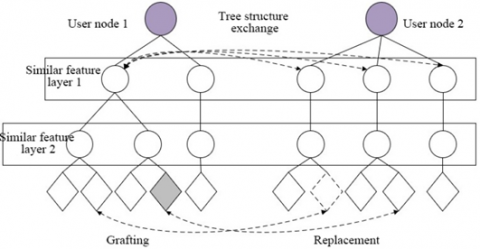Figure 2. The hierarchical pyramid-tree

The redundancy of multidimensional mobile crowd sensing data can be identified through multi-feature clustering under the semantic constraints. To discover the redundant or missing data of user nodes at the semantic level, the tree structures of two nodes must be exchanged at the moment of contact. The missing items need to be grafted, and the redundant items, replaced with high-quality data.

3. Data Collection Strategy

The core issues in the application of mobile crowd sensing are properly evaluating data quality and treating redundant items. Using an observation matrix unrelated to the transformation base, compressed crowd sensing maps the compressible or sparse high-dimensional data in the transformation domain to a low-dimensional space. In this way, the reconstruction of the original data becomes an optimization problem.

To improve the quality of sensing data and reduce the sensing cost, this paper proposes a compressed mobile crowd sensing method based on tensor completion and sparse sampling, in view of the high spatiotemporal correlations between mobile terminal outputs.

3.1 Construction and solution of tensor completion model

The mathematical model of tensor compaction can be expressed as:

$\underset{Q}{\mathop{\min }}\,rank(Q)\text{ }s.t.{{Q}_{\Phi }}={{A}_{\Phi }}$     (1)

where, rank(Q) is the rank of the tensor. The tensor rank can be defined as Tucker rank (i.e. n-rank) or CANDECOMP/PARAFAC (CP) rank (CANDECOMP: canonical decomposition; PARAFAC: parallel factors decomposition). Here, the Tucker rank definition is selected. Let qi be the mode-n expansion of tensor Q. Then, the model optimization problem can be expressed as:

$\left\{ \begin{matrix} \underset{Q}{\mathop{\min }}\,\sum\limits_{i=1}^{N}{rank\left( {{q}_{i}} \right)} \\ s.t.{{Q}_{\Phi }}={{A}_{\Phi }} \\\end{matrix} \right.$     (2)

Tensor completion can be solved in two paths: (1) Minimize the tensor trace norm, i.e., transform the low-rank tensor completion problem into a low-rank matrix completion problem by mapping high-dimensional tensor into matrices. (2) Optimize tensor decomposition, i.e., solve low-rank tensor completion by the idea of tensor algebra; The options include singular value decomposition (SVD), CP decomposition (Figure 3), and Tucker decomposition (Figure 4).

## 3.pngFigure 3. The CP decomposition

## 4.png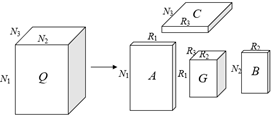Figure 4. The Tucker decomposition

For path 1, the optimization problem of tensor completion can be transformed with the following tensor trace norm:

$\left\{ \begin{matrix} \text{min}\frac{1}{2}{{\left\| Q-A \right\|}_{H}}+\sum\limits_{i=1}^{3}{{{\omega }_{i}}}{{\left\| {{q}_{i}} \right\|}_{*}} \\ s.t.{{Q}_{\Phi }}={{A}_{\Phi }} \\\end{matrix} \right.$     (3)

where, ‖qi* is the kernel norm of each matrix after tensor expansion; ωi is the weighting coefficient of the kernel norm of each matrix. Suppose the number of kernel norms equals the sum of singular values. Then, the SVD with trace norm can be expressed as:

$\left\{ \begin{matrix} \text{min}\frac{1}{2}{{\left\| Q-A \right\|}_{H}}+\sum\limits_{i=1}^{3}{{{\omega }_{i}}}{{\left\| \text{S}VD\left( {{q}_{i}} \right) \right\|}_{\text{0}}} \\ s.t.{{Q}_{\Phi }}={{A}_{\Phi }} \\\end{matrix} \right.$     (4)

For path 2, the general mathematical model for tensor completion can be expressed as:

$\underset{Q}{\mathop{\text{min}}}\,\frac{1}{2}{{\left\| Z*\left( Q-A \right) \right\|}_{H}}$     (5)

where, Z is the indicator tensor, which sets the unknown positions of matrix elements to zeros and the known positions to ones. Through CP decomposition of tensor Q, the optimization problem can be corrected as:

\begin{align} & CP(A,B,C)= \\ & \frac{1}{2}{{\left\| Z*\left( Q-ABC \right) \right\|}^{2}}+\eta \left( {{\left\| \text{A} \right\|}^{2}}+{{\left\| \text{B} \right\|}^{2}}+{{\left\| \text{C} \right\|}^{2}} \right) \\\end{align}     (6)

Through Tucker decomposition of tensor Q, the optimization problem can be converted into:

\begin{align} & f(W,A,B,C)= \\ & \arg \min \frac{1}{2}\left\| Z*(Q-{{S}_{\times 1}}{{A}_{\times 2}}{{B}_{\times 3}}C \right\|_{H}^{2} \\\end{align}     (7)

3.2 Data collection through compressed crowd sensing based on sparse sampling

The key to reconstructing the original data is the random sparse sampling of low-rank tensors. Our random sparse sampling method takes basis on theory of compressed crowd sensing. For any data sequence c, the sparse vector c*=ΓTc obtained by orthogonal basis Γ transform is sparse if it satisfies:

${{\left\| {{c}^{*}} \right\|}_{r}}={{\left( \sum\limits_{i}{{{\left| c_{i}^{*} \right|}^{r}}} \right)}^{1/r}}\le P$      (8)

where, $r \in$[0, 2]; P>2. If the transformation coefficients of the data sequence decay exponentially under the orthogonal basis Γ, then all terms with close-to-zero coefficients can be neglected. In this case, the sparse approximation of data sequence c can be achieved with the linear combination of a few terms with large coefficients. Here, the sparsity of the linear combination is set to k, that is, the k basis vectors with nonzero coefficients of orthogonal basis Γ. At this time, the sparse vector c* has M-k coefficients equal to zero (kM). Then, the data sequence c can be expressed:

$c=\Gamma {{c}^{*}}$     (9)

Based on compressed sensing theory, the original data are reconstructed with the compressed N-dimensional signal, rather than the original M-dimensional signal. Let Φ be an N×M matrix of linear measurement. Then, the mathematical model of linear measurement L can be expressed as:

$L=\Psi c=\Psi \Gamma {{c}^{*}}=\Phi {{c}^{*}}$     (10)

The original data can be reconstructed by extracting the N-dimensional data sequence from the measurement L=Ψc. The reconstruction can be completed by solving (10), that is, the optimization problem (11):

${{\hat{c}}^{*}}=\arg \min {{\left\| {{c}^{*}} \right\|}_{0}}y=\Psi \Gamma {{c}^{*}}$     (11)

As can be seen from (13), solving one L0-norm optimization problem is sufficient to recover c from the sparsely sampled data sequence. However, this problem is rather complex to solve directly, and often transformed into an L1-norm optimization problem. Being the most important matrix of compressed crowd sensing, the linear measurement matrix Φ must have the restricted isometry property (RIP):

$(1-{{\lambda }_{d}})\left\| y \right\|_{2}^{2}\le \left\| {{x}_{d}}y \right\|_{2}^{2}\le (1+{{\lambda }_{d}})\left\| y \right\|_{2}^{2}$     (12)

That is, if 1<d<b and d are integers, there exists a λd(0<λd<1) making each a×d submatrix Xd and every vector y of a×b matrix X satisfy (12).

The case where the measurement matrix Ψ is not correlated with sparse orthogonal basis matrix Γ is equivalent to the RIP criterion. Let Ψi denote a vector of Ψ, and Γi denote a vector of Γ. The correlation coefficient can be defined as:

$\varphi=\max _{i^{*} j}\left|\Psi_{i}, \Gamma_{i}\right|$    (13)

where, φ>0 describes the correlation between Ψ and Γ. The φ value is positively correlated with the degree of correlation between the two matrices.

In the traditional theory on compressed crowd sensing, the target data sequence is usually discrete. Hence, the compressed sensing framework should be expanded to suit the collection of continuous analog signals in actual application. For this purpose, an analog signal converter was designed (Figure 5).

## 5.png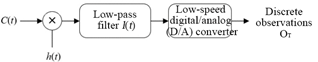Figure 5. The analog signal converter

Let C(t) be an analog signal with a finite information rate, composed of a limited number of discrete weighted continuous bases:

$C(t)=\sum\limits_{n=1}^{N}{{{\beta }_{n}}}{{\gamma }_{n}}(t)$     (14)

In the light of Figure 2, the discrete observations can be expressed as:

\begin{align} & {{o}_{T}}=\int{_{-\infty }^{+\infty }}C(\varepsilon )h(\varepsilon )l(t-\varepsilon )d\tau {{|}_{t=T}} \\ & =\text{ }\int{_{-\infty }^{+\infty }}\sum\limits_{n-1}^{N}{{{g}_{n}}\gamma }(\tau )h(\tau )l(t-\tau )d\tau {{|}_{t=T}} \\ & =\text{ }\sum\limits_{n-1}^{N}{{{g}_{n}}\int{_{-\infty }^{+\infty }}\gamma }(\tau )h(\tau )l(T-\tau )d\tau \\\end{align}     (15)

That is:

$o=\sum\limits_{n-1}^{N}{{{\beta }_{n}}}{{K}_{T,n}}=\Phi {{c}^{*}}$     (16)

where,

${{K}_{T,n}}=\int{_{-\theta }^{+\theta }}{{\gamma }_{n}}(\tau )h(\tau )l(T-\tau )d\tau$     (17)

By (17), the matrix Φ of the reconstruction algorithm can be established, laying the basis for effective reconstruction of the sampling data.

3.3 Adaptive data collection strategy based on tensor completion

In this research, the items of mobile crowd sensing data are defined as the real-time temperatures in a city. Firstly, the urban area D was meshed into S1×S2 grids at a certain interval d. The center of each grid is the sampling point of the real-time temperature, denoted as (si, sj), i=1, …, S1, j=, …, S2. During data collection, the users measure the temperatures of T hours with mobile phone or other intelligent mobile terminals. The tensor of real-time temperatures in the 2D area D was defined as a third-order tensor formed by the temperatures of T hours at any sampling point (s1, s2), denoted as Tem(s1, s2, t)$\in$RSST.

Let n be the preset number of samples, which is much smaller than S1×S2. Then, temperatures were collected from n out of all sampling points. The goal of data collection is to reconstruct the complete tensor of real-time temperatures through sparse sampling in urban area D, that is, reconstruct Tem from the data sampled by n users. After data collection, the measurement matrix L can be obtained as:

$L={{\nabla }_{\Phi }}\text{(}Tem\text{)}$     (18)

where, $\nabla_{\Phi}(A)$ is the sampling operator. If (s1, s2)$\in \Phi$, then L(s1, s2, t)=Tem(s1, s2, t); otherwise, L(s1, s2, t) is 0.

To reduce the sensing cost, the preset number of samples could only cover a subset of all sampling points, with the hope that the original tensor Tem can be fully recovered from the observation tensor L.

Before tensor completion of the collected real-time temperatures, it must be ensured that the target tensor Tem is approximately low-rank. To solve the approximate tensor Tem*, the tensor rank should be minimized, and then the restored approximate tensor Tem* should be kept equal to the observation tensor L at the sampling points. Then, it is a must to solve the following optimization problem:

$\left\{ \begin{matrix} \left\langle Te{{m}^{*}},\Phi \right\rangle =\underset{Te{{m}^{*}},\Phi }{\mathop{\text{argmin}}}\,\left\| {{\nabla }_{\Phi }}(L-Te{{m}^{*}}) \right\|_{H}^{2}+\delta \cdot rank(Te{{m}^{*}}) \\ s.t.\text{ }\left| \Phi \right|\le n \\\end{matrix} \right.$     (19)

where, δ is the parameter to adjust the ratio of accurate fitting to minimization of tensor rank.

In the above model, the set Φ of sampling point positions and the approximate tensor Tem* are both uncertain, and both include two objectives: sampling and reconstruction. In other words, it is attempted to build a set Φ of samples L that can contain most of the information in the original data Tem, and estimate the complete original tensor Tem from the observation tensor L, such as to minimize the error between the estimated value Tem* and the original data Tem.

After the construction of set Φ, finding the rank is a problem with non-deterministic polynomial-time (NP) hardness. Thus, it is an NP-hard problem to solve the optimization problem (19). Replacing rank(Tem*) with ||Tem*||, (19) can be transformed into:

$Te{{m}^{*}}=\underset{Te{{m}^{*}}}{\mathop{\arg \min }}\,\left\| {{\nabla }_{\Phi }}\left( L-Te{{m}^{*}} \right) \right\|_{H}^{2}+\delta \left\| Te{{m}^{*}} \right\|$     (20)

3.4 Algorithm implementation

Based on tensor completion and sparse sampling, our strategy of compressed mobile crowd sensing relies on adaptability to identify the highly informative elements for the low-dimensional tensor subspaces of real-time temperatures in the urban area. The data collection can be divided into two phases: collecting the basic information of urban area D; sampling at the points with rich information. The total present number of samples n can be split into ρn and (1-ρ)n for the two phases by the ratio ρ.

The workflow of our algorithm goes as follows:

Step 1. Initialization

Initialize tensor dimensions S1 and S2, T, ratio ρ, and number of iterations M.

Step 2. Horizontal sparse sampling

Perform horizontal sampling at ρn/S2 points in urban area D uniformly, allocate the samples collected by the i-th sampling to the set Φ1j, and establish the sample set Φ1 of phase 1 after S2 samplings.

Step 3. Vertical iterative sampling

Estimate the sampling frequency, allocate the remaining (1-ρ)n samples to the high-information columns identified in phase 1, according to the distribution of sampling frequency, and form the set Φj of vertical samples after the j-th iteration.

Step 4. Tensor reconstruction

Approximate the temperature Tem* at unsampled points based on the sampled value Tem.

4. Experiments and Result Analysis

The original data were collected through crowd sensing from the area of a city within the Third Ring Road. The target area was evenly meshed into 50*50=2,500 grids. The missing items in the collected data were supplemented by the k-nearest neighbors (k-NN) algorithm.

To fully disclose the features of real-time temperature in the city, the data were collected from multiple time intervals and time periods, and the coherence and singular values of the tensor of sensing data were analyzed in details.

Figure 6 provides the row- and column-space coherence curves of different time intervals. It can be seen that the distribution of row-space/column-space coherences varied with time intervals. The two parameters were not distributed uniformly, during the periods with drastic temperature changes; that is, the temperature distribution is uneven. The two parameters were distributed evenly, during the periods with slight temperature changes; that is, the temperature distribution is even. Figure 7 presents the singular value distribution of the tensor norm.

## 61.png(a)

## 62.png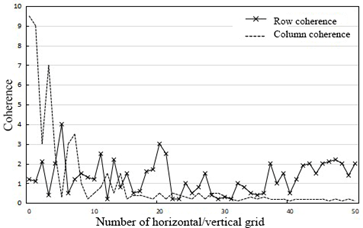(b)

Figure 6. The row- and column-space coherence curves of different time intervals

## 7.png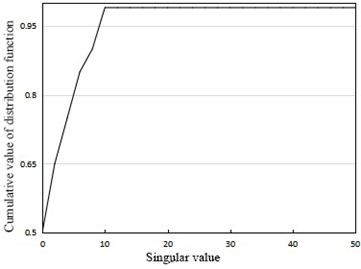Figure 7. The singular value distribution of the tensor norm

## 81.png(a)

## 82.png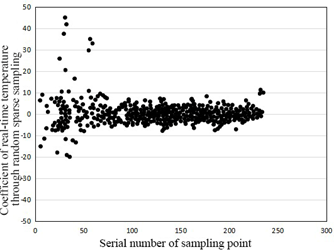(b)

Figure 8. The temperature changes of the city through random sparse sampling

## 9.png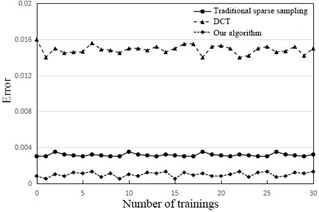Figure 9. The relative errors of three sampling algorithms in data recovery

## 101.png(a)

## 102.png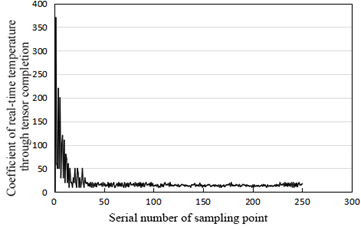(b)

Figure 10. The temperature changes through tensor completion

Next, contrastive experiments were carried out to verify the effectiveness of the proposed random sparse sampling method in compressed crowd sensing. The temperature changes of the city through random sparse sampling are recorded in Figure 8, where the abscissas in subgraphs (a) and (b) are both the serial number of sampling point; the ordinate in subgraph (a) is the temperature; the ordinate in subgraph (b) is the temperature coefficient obtained from the corresponding point through random sparse sampling. It can be seen that the temperatures obtained through compressed crowd sensing were orderly, compact, and regular.

Furthermore, the proposed algorithm was compared with traditional sparse sampling, and discrete cosine transform (DCT). Figure 9 compares the relative errors of the three methods in data recovery. Obviously, our algorithm achieved the lowest error, an evidence to its superiority in data reconstruction.

## 111.png(a)

## 112.png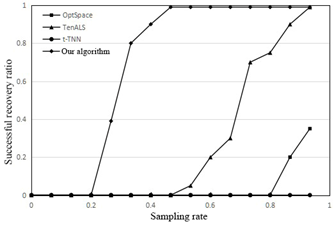(b)

Figure 11. The relative errors and successful recovery ratios of crowd sensing temperatures

Figure 10 records the temperature changes through tensor completion, where the abscissas and ordinates are similar to those of Figure 8. It is easy to learn that tensor completion reduced the number of nonzero temperature coefficients, leaving only a few large positive values (the first few coefficients). This means compressed crowd sensing can effectively reduce the number of sampling data, and simplify the calculation, while ensuring the restoration of the original data.

Finally, our algorithm was tested on an actual air quality dataset, in comparison with the OptSpace algorithm, tensor alternating least squares (TenALS), and twist tensor nuclear norm (t-TNN). The two subgraphs of Figure 11 compare the relative errors and successful recovery ratios of the four methods, respectively. It can be seen that our algorithm clearly outperformed the three contrastive methods. When the sampling rate was greater than 45%, our algorithm could accurately reconstruct all the sensing data.

5. Conclusions

To maximize the accuracy of mobile crowd sensing system, this paper presents a novel data collection method based on tensor completion and sparse sampling. Firstly, the architecture of mobile crowd sensing system was designed in the context of big data, and the principle of data optimization was explained in details. Next, an adaptive collection method was developed for mobile crowd sensing data in sparse form or in the form of 3D tensor. Finally, the coherence and singular values of the tensor of urban temperatures were analyzed, and contrastive experiments were conducted to prove that our method is effective in compressed crowed sensing and accurate in data reconstruction.

Acknowledgment

This work is supported by National Natural Science Foundation of China Grant (Grant No.: 11626080) and Natural Science Foundation of Hebei Province (Grant No.: A2017207011).

References

 Wang, W., Aggarwal, V., Aeron, S. (2016). Tensor completion by alternating minimization under the tensor train (TT) model. arXiv preprint arXiv:1609.05587. https://arxiv.org/abs/1609.05587

 Ko, C.Y., Batselier, K., Daniel, L., Yu, W., Wong, N. (2020). Fast and accurate tensor completion with total variation regularized tensor trains. IEEE Transactions on Image Processing, 29: 6918-6931. https://doi.org/10.1109/TIP.2020.2995061

 Steinlechner, M. (2016). Riemannian optimization for high-dimensional tensor completion. SIAM Journal on Scientific Computing, 38(5): S461-S484. https://doi.org/10.1137/15M1010506

 Da Silva, C., Herrmann, F.J. (2015). Optimization on the hierarchical tucker manifold–applications to tensor completion. Linear Algebra and its Applications, 481: 131-173. https://doi.org/10.1016/j.laa.2015.04.015

 Kasai, H., Mishra, B. (2016). Low-rank tensor completion: A Riemannian manifold preconditioning approach. In International Conference on Machine Learning, pp. 1012-1021.

 Madathil, B., George, S.N. (2018). Twist tensor total variation regularized-reweighted nuclear norm based tensor completion for video missing area recovery. Information Sciences, 423: 376-397. https://doi.org/10.1016/j.ins.2017.09.058

 Filipović, M., Jukić, A. (2015). Tucker factorization with missing data with application to low-n-rank tensor completion. Multidimensional Systems and Signal Processing, 26: 677-692. https://doi.org/10.1007/s11045-013-0269-9

 Yokota, T., Zhao, Q., Cichocki, A. (2016). Smooth PARAFAC decomposition for tensor completion. IEEE Transactions on Signal Processing, 64(20): 5423-5436. https://doi.org/10.1109/TSP.2016.2586759

 Song, Q., Ge, H., Caverlee, J., Hu, X. (2019). Tensor completion algorithms in big data analytics. ACM Transactions on Knowledge Discovery from Data (TKDD), 13(1): 1-48. https://doi.org/10.1145/3278607

 Liu, C., Shan, H., Chen, C. (2020). Tensor p-shrinkage nuclear norm for low-rank tensor completion. Neurocomputing, 387: 255-267. https://doi.org/10.1016/j.neucom.2020.01.009

 Yokota, T., Zhao, Q., Cichocki, A. (2016). Smooth PARAFAC decomposition for tensor completion. IEEE Transactions on Signal Processing, 64(20): 5423-5436. https://doi.org/10.1109/TSP.2016.2586759

 Zhou, P., Lu, C., Lin, Z., Zhang, C. (2017). Tensor factorization for low-rank tensor completion. IEEE Transactions on Image Processing, 27(3): 1152-1163. https://doi.org/10.1109/TIP.2017.2762595

 Guo, B., Liu, Y., Wu, W., Yu, Z., Han, Q. (2016). Activecrowd: A framework for optimized multitask allocation in mobile crowdsensing systems. IEEE Transactions on Human-Machine Systems, 47(3): 392-403. https://doi.org/10.1109/THMS.2016.2599489

 Capponi, A., Fiandrino, C., Kantarci, B., Foschini, L., Kliazovich, D., Bouvry, P. (2019). A survey on mobile crowdsensing systems: Challenges, solutions, and opportunities. IEEE Communications Surveys & Tutorials, 21(3): 2419-2465. https://doi.org/10.1109/COMST.2019.2914030

 Piao, C., Liu, C.H. (2019). Energy-efficient mobile crowdsensing by unmanned vehicles: A sequential deep reinforcement learning approach. IEEE Internet of Things Journal, 7(7): 6312-6324. https://doi.org/10.1109/JIOT.2019.2962545

 Xiao, L., Chen, T., Xie, C., Dai, H., Poor, H.V. (2017). Mobile crowdsensing games in vehicular networks. IEEE Transactions on Vehicular Technology, 67(2): 1535-1545. https://doi.org/10.1109/TVT.2016.2647624

 Wang, L., Zhang, D., Xiong, H., Gibson, J. P., Chen, C., Xie, B. (2016). ecoSense: Minimize participants’ total 3G data cost in mobile crowdsensing using opportunistic relays. IEEE Transactions on Systems, Man, and Cybernetics: Systems, 47(6): 965-978. https://doi.org/10.1109/TSMC.2016.2523902

 Karaliopoulos, M., Telelis, O., Koutsopoulos, I. (2015). User recruitment for mobile crowdsensing over opportunistic networks. In 2015 IEEE Conference on Computer Communications (INFOCOM), Kowloon, Hong Kong, pp. 2254-2262. https://doi.org/10.1109/INFOCOM.2015.7218612

 Capponi, A., Fiandrino, C., Kliazovich, D., Bouvry, P. (2017). Energy efficient data collection in opportunistic mobile crowdsensing architectures for smart cities. In 2017 IEEE Conference on Computer Communications Workshops (INFOCOM WKSHPS), pp. 307-312. https://doi.org/10.1109/INFCOMW.2017.8116394

 Lane, N.D., Miluzzo, E., Lu, H., Peebles, D., Choudhury, T., Campbell, A.T. (2010). A survey of mobile phone sensing. IEEE Communications Magazine, 48(9): 140-150. https://doi.org/10.1109/MCOM.2010.5560598

 Karaliopoulos, M., Koutsopoulos, I., Spiliopoulos, L. (2019). Optimal user choice engineering in mobile crowdsensing with bounded rational users. In IEEE INFOCOM 2019-IEEE Conference on Computer Communications, pp. 1054-1062. https://doi.org/10.1109/INFOCOM.2019.8737386

 Karaliopoulos, M., Koutsopoulos, I., Titsias, M. (2016). First learn then earn: Optimizing mobile crowdsensing campaigns through data-driven user profiling. In Proceedings of the 17th ACM International Symposium on Mobile Ad Hoc Networking and Computing, pp. 271-280. https://doi.org/10.1145/2942358.2942369

 Fiandrino, C., Anjomshoa, F., Kantarci, B., Kliazovich, D., Bouvry, P., Matthews, J.N. (2017). Sociability-driven framework for data acquisition in mobile crowdsensing over fog computing platforms for smart cities. IEEE Transactions on Sustainable Computing, 2(4): 345-358. https://doi.org/10.1109/TSUSC.2017.2702060

 Liu, J., Shen, H., Narman, H.S., Chung, W., Lin, Z. (2018). A survey of mobile crowdsensing techniques: A critical component for the internet of things. ACM Transactions on Cyber-Physical Systems, 2(3): 1-26. https://doi.org/10.1145/3185504

 Capponi, A., Fiandrino, C., Kantarci, B., Foschini, L., Kliazovich, D., Bouvry, P. (2019). A survey on mobile crowdsensing systems: Challenges, solutions, and opportunities. IEEE Communications Surveys & Tutorials, 21(3): 2419-2465. https://doi.org/10.1109/COMST.2019.2914030